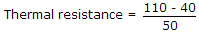# Electronics and Communication Engineering - Power Electronics

### Exercise :: Power Electronics - Section 3

31.

In a semi converter feeding a resistive load, the firing angle is a. Each thyristor conducts for

 A. p radians B. (p - a) radians C. (p + a) radians D. (p - 0.5 a) radians

Explanation:

The conduction is from a to p.

32.

Between which points does UJT exhibit negative resistance characteristics

 A. between peak and valley points B. before peak point C. after peak point D. after valley point

Explanation:

No answer description available for this question. Let us discuss.

33.

A thyristor has maximum allowable junction temperature of 110° C and the ambient temperature is 40°C, If the maximum internal power dissipation is 50 W, the thermal resistance is

 A. 2° C/W B. 1.4° C/W C. 0.7°C/W D. 2.2° C/W

Explanation:.

34.

A single phase electric heater uses phase controlled thyristors. The firing angle is 90°. The degree of heating is

 A. 25% of maximum B. 50% of maximum C. 75% of maximum D. none of the above

Explanation:

Conduction occurs only during 50% of wave.

35.

Which of these commutation methods is used in AC-DC converters?

 A. Class A B. Class C C. Class D D. Class F